# Chemical Engineering- CH 2013 GATE Paper (Practice Test)

## 65 Questions MCQ Test GATE Past Year Papers for Practice (All Branches) | Chemical Engineering- CH 2013 GATE Paper (Practice Test)

Description
Attempt Chemical Engineering- CH 2013 GATE Paper (Practice Test) | 65 questions in 180 minutes | Mock test for GATE preparation | Free important questions MCQ to study GATE Past Year Papers for Practice (All Branches) for GATE Exam | Download free PDF with solutions
QUESTION: 1

### Q. No. 1 – 5 Carry One Mark Each Q. If 3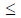X5 and 8Y11 then which of the following options is TRUE?

Solution: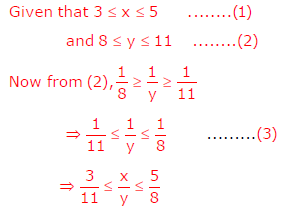QUESTION: 2

Solution:
QUESTION: 3

### Mahatama Gandhi was known for his humility as

Solution:

P and R are true

QUESTION: 4

"All engineering students" "should learn mechanics", "mathematics and" "how to do computation."
I                                          II                                  III                              IV
Which of the above underlined parts of the sentence is not appropriate?

Solution:
QUESTION: 5

Select the pair that best expresses a relationship similar to that expressed in the
pair:

water: pipe::

Solution:
QUESTION: 6

Q. No. 6 – 10 Carry Two Marks Each

Q.

Velocity of an object fired directly in upward direction is given by V = 80 − 32 t,
where t (time) is in seconds. When will the velocity be between 32 m/sec and 64
m/sec?

Solution:
QUESTION: 7

In a factory, two machines M1 and M2 manufacture 60% and 40% of the autocomponents respectively. Out of the total production, 2% of M1 and 3% of M2 are found to be defective. If a randomly drawn autocomponent from the combined lot is found defective, what is the probability that it was manufactured
by M2?

Solution:

Let E1 be the machine M1 manufacture auto companies
Let E2 be the event of machine M2 manufacture auto companies
Given P(E1 )= 60%  = 60/100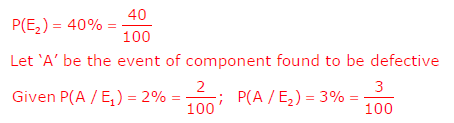A randomly draw on auto component from the lot is sound defective the
probability it was manufactured by M2 is  P(E2 / A) we have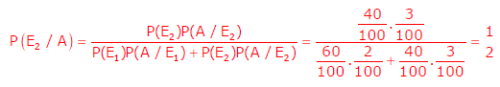QUESTION: 8

Following table gives data on tourists from different countries visiting India in the
year 2011.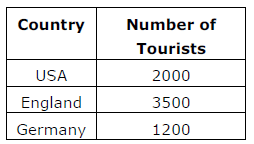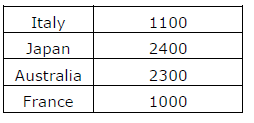Which two countries contributed to the one third of the total number of tourists
who visited India in 2011?

Solution:
QUESTION: 9

If |−2X + 9| = 3 then the possible value of |−X| − X2 would be:

Solution: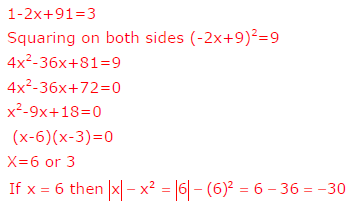QUESTION: 10

All professors are researchers
Some scientists are professors
Which of the given conclusions is logically valid and is inferred from the above
arguments:

Solution:
QUESTION: 11

Q. No. 11 – 25 Carry One Mark Each

Q.

The number of emails received on six consecutive days is 11, 9, 18, 18, 4 and 15,
respectively.

What are the median and the mode for these data?

Solution:

By arranging the data in ascending order, the data is 4, 9, 11, 15, 18, 18
Median  =(15+11)/2=13
Mode=Maximum number of times occurred value =18

*Answer can only contain numeric values
QUESTION: 12

For two rolls of a fair die, the probability of getting a 4 in the first roll and a
number less than 4 in the second roll, up to 3 digits after the decimal point, is _______

Imp : you should answer only the numeric value

Solution:

We known that, if two dice are thrown then total number of out comes
=62=39
Let E be the event of getting 4 in the first roll and a number less than 4 is the
second.
Then favorable cases to occur E are

(4,1) (4,2), (4,3)

Re quired probability =3/36=1/12 =0.0833

QUESTION: 13

Which of the following statements are TRUE?
P. The eigen values of a symmetric matrix are real
Q. The value of the determinant of an orthogonal matrix can only be +1
R. The transpose of a square matrix A has the same eigen values as those of A
S. The inverse of an ‘n × n’ matrix exists if and only if the rank is less than ‘n’

Solution:

P and R are true

QUESTION: 14

Evaluate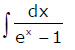(Note: C is a constant of integration)

Solution: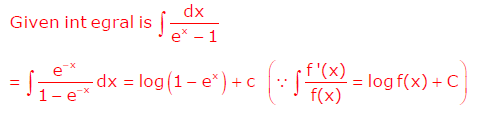*Answer can only contain numeric values
QUESTION: 15

A gaseous system contains H2, I2, and HI, which participate in the gas-phase
reaction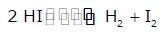At a state of reaction equilibrium, the number of thermodynamic degrees of
freedom is

Imp : you should answer only the numeric value

Solution:

As we know F = C - P+ 2
Here C = 2, P = 1 so F= 2- 1+ 2
F = 3

QUESTION: 16

The thermodynamic state of a closed system containing a pure fluid changes from
(T1, p1) to (T2, p2), where T and p denote the temperature and pressure,
respectively. Let Q denote the heat absorbed (> 0 if absorbed by the system) and
W the work done (> 0 if done by the system). Neglect changes in kinetic and
potential energies. Which one of the following is CORRECT?

Solution:

C
Q −W = ΔU
ΔU which solely depends only on initial and final temperature. It is independent
of path.

QUESTION: 17

An equation of state is explicit in pressure p and cubic in the specific volume v. At
the critical point ‘c’, the isotherm passing through ‘c’ satisfies

Solution: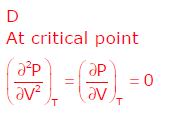QUESTION: 18

The units of the isothermal compressibility are

Solution: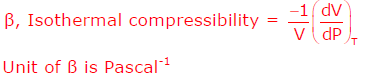*Answer can only contain numeric values
QUESTION: 19

An open tank contains two immiscible liquids of densities (800 kg/m3 and 1000 kg/m3) as shown in the figure. If g = 10 m/s2, under static conditions, the gauge pressure at the bottom of the tank in Pa is _____________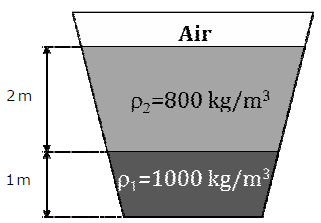Important : you should answer only the numeric value

Solution: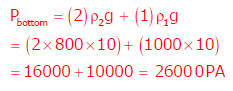QUESTION: 20

The apparent viscosity of a fluid is given by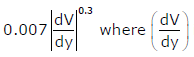is the velocity gradient. The fluid is

Solution: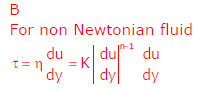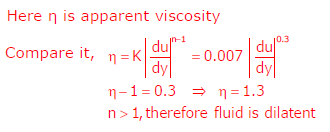QUESTION: 21

The mass balance for a fluid with density (ρ) and velocity vector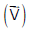is

Solution: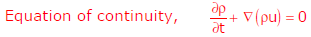QUESTION: 22

An incompressible Newtonian fluid, filled in an annular gap between two concentric cylinders of radii R1 and R2 as shown in the figure, is flowing under steady state conditions. The outer cylinder is rotating with an angular velocity of ? while the inner cylinder is stationary. Given that ( R1-R2)  ? R1 the profile of the θ -component of the velocity Vθ can be approximated by,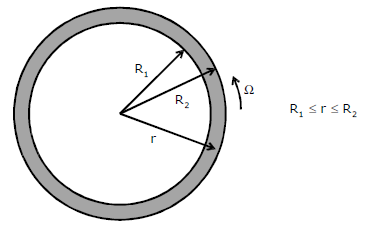Solution:
QUESTION: 23

For a Newtonian fluid flowing in a circular pipe under steady state conditions in
fully developed laminar flow, the Fanning friction factor is

Solution: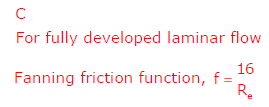QUESTION: 24

In the Tyler standard screen scale series, when the mesh number increases from
3 mesh to 10 mesh, then

Solution: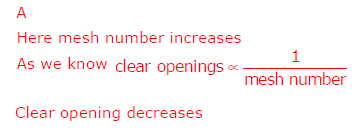*Answer can only contain numeric values
QUESTION: 25

Taking the acceleration due to gravity to be 10 m/s2, the separation factor of a cyclone 0.5 m in diameter and having a tangential velocity of 20 m/s near the wall is_____

Important : you should answer only the numeric value

Solution: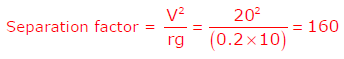QUESTION: 26

The effectiveness of a heat exchanger in the ε − NTU method is defined as

Solution: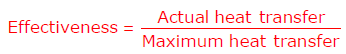QUESTION: 27

In a pool boiling experiment, the following phenomena were observed.
P. Natural convection
Q. Film boiling
R. Transition boiling
S. Nucleate boiling
What was the CORRECT sequence of their occurrence?

Solution:

Sequence is
(P) Natural convection    (S) Nucleate convection
(R) Transition boiling       (Q) Film boiling

*Answer can only contain numeric values
QUESTION: 28

A hole of area 1 cm2 is opened on the surface of a large spherical cavity whose inside temperature is maintained at 727 °C. The value of Stefan-Boltzmann constant is 5.67×10-8 W/m2-K4. Assuming black body radiation, the rate at which the energy is emitted (in W) by the cavity through the hole, up to 3 digits after the decimal point, is __________

Important : you should answer only the numeric value

Solution: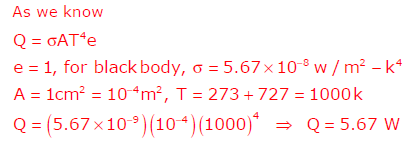QUESTION: 29

The packing of an existing absorption tower is replaced with a new type of packing. The height of the packing and the inlet conditions are maintained the same as before. Tests reveal that the number of transfer units is lower than before. This indicates that the tower with the new packing, when compared to
that with the old packing, will

Solution:

Here NTU is lowered which suggests that new packing have a lower rate of
absorption of solute from the gas stream in comparison to old packing.

QUESTION: 30

A wet solid is dried over a long period of time by unsaturated air of nonzero constant relative humidity. The moisture content eventually attained by the solid is termed as the

Solution:

Equilibrium moisture is attained by the solid after drying over a long period of
time.

*Answer can only contain numeric values
QUESTION: 31

The exit age distribution for a reactor is given by E(t) = δ(t − 4), where t is in seconds. A first order liquid phase reaction (k = 0.25 s-1) is carried out in this reactor under steady state and isothermal conditions. The mean conversion of the reactant at the exit of the reactor, up to 2 digits after the decimal point, is

Important : you should answer only the numeric value

Solution:

General equation for first order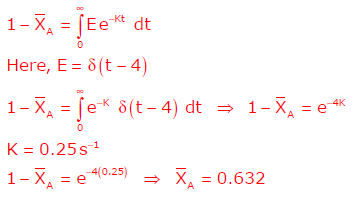*Answer can only contain numeric values
QUESTION: 32

An isothermal liquid phase zero order reaction A→B (k = 0.5 mol/m3-s) is carried out in a batch reactor. The initial concentration of A is 2 mol/m3. At 3 seconds from the start of the reaction, the concentration of A in mol/m3 is

Important : you should answer only the numeric value

Solution: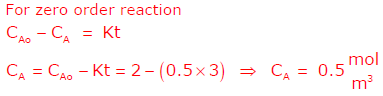*Answer can only contain numeric values
QUESTION: 33

The overall rates of an isothermal catalytic reaction using spherical catalyst particles of diameters 1 mm and 2 mm are rA1 and rA2 (in mol (kg-catalyst)-1 h-1), respectively. The other physical properties of the catalyst particles are identical. If pore diffusion resistance is very high, the ratio rA2/rA1 is

Important : you should answer only the numeric value

Solution: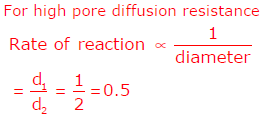QUESTION: 34

In the manufacture of sulphuric acid by the contact process, the catalytic
oxidation of SO2 is carried out in multiple stages mainly to

Solution:

C

To increase the overall conversion interstage cooling is provided.

QUESTION: 35

Match the following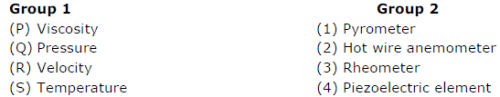Solution: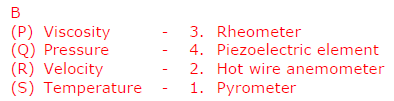(P)-3, (Q)-4, (R)-2, (S)-1

*Answer can only contain numeric values
QUESTION: 36

Q. No. 36 – 65 Carry Two Marks Each

For the function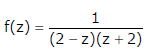The residue at z=2 is___________

Solution: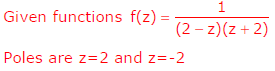Required answer is residue at z=2
Z=2 is a simple pole, therefore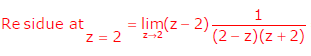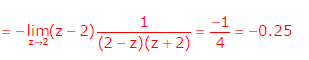QUESTION: 37

The solution of the differential equation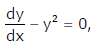given y = 1 at x= 0 is

Solution: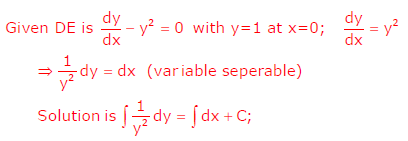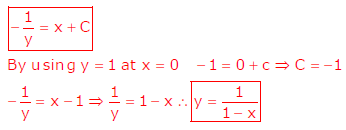QUESTION: 38

The solution of the differential equation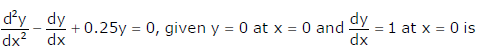Solution: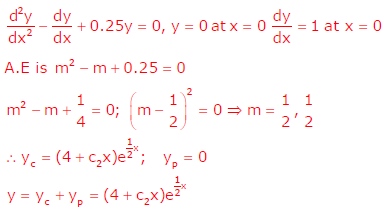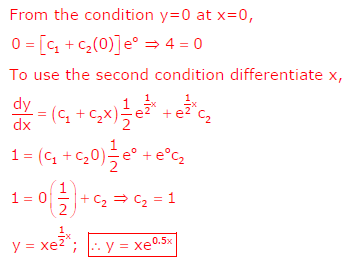*Answer can only contain numeric values
QUESTION: 39

The value of the integral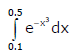evaluated by Simpson’s rule using 4 subintervals (up to 3 digits after the decimal
point) is______

Important : you should answer only the numeric value

Solution: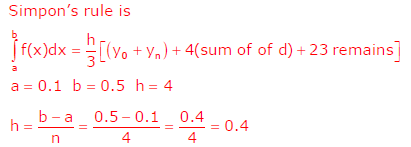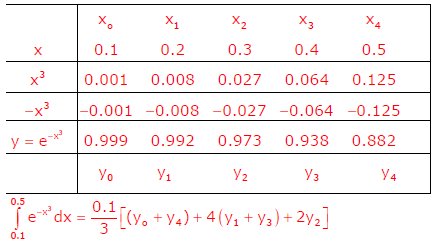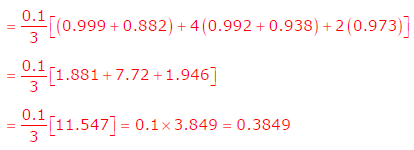QUESTION: 40

In a process occurring in a closed system F, the heat transferred from F to the surroundings E is 600 J. If the temperature of E is 300 K and that of F is in the range 380 - 400 K, the entropy changes of the surroundings (SSE) and system (ΔSF), in J/K, are given by

Solution: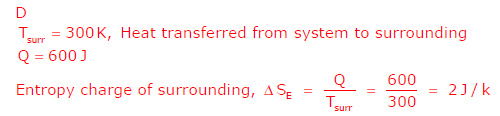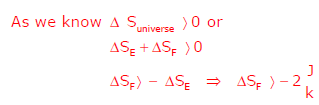*Answer can only contain numeric values
QUESTION: 41

A binary liquid mixture is in equilibrium with its vapor at a temperature T = 300 K. The liquid mole fraction x1 of species 1 is 0.4 and the molar excess Gibbs free energy is 200 J/mol. The value of the universal gas constant is 8.314 J/mol-K, and γi  denotes the liquid-phase activity coefficient of species i. If ln ( γi ) = 0.09,
then the value of ln( γ2 ), up to 2 digits after the decimal point, is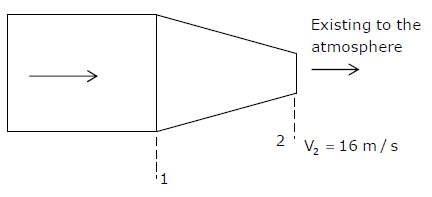Important : you should answer only the numeric value

Solution: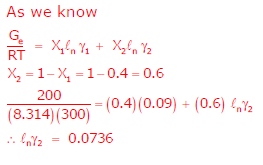*Answer can only contain numeric values
QUESTION: 42

Water (density 1000 kg/m3) is flowing through a nozzle, as shown below and exiting to the atmosphere. The relationship between the diameters of the nozzle at locations 1 and 2 is D1 = 4 D2. The average velocity of the stream at location 2 is 16 m/s and the frictional loss between location 1 and location 2 is 10000 Pa.
Assuming steady state and turbulent flow, the gauge pressure in Pa, at location 1
is _________

Important : you should answer only the numeric value

Solution: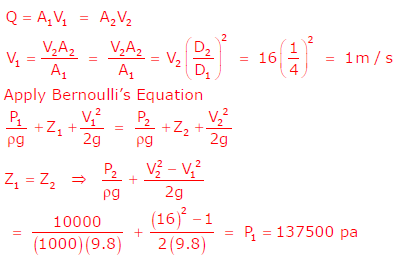QUESTION: 43

In the elutriation leg of a commercial crystallizer containing a mixture of coarse and very fine crystals of the same material, a liquid is pumped vertically upward. The liquid velocity is adjusted such that it is slightly lower than the terminal velocity of the coarse crystals only. Hence

Solution:

If velocity 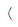Terminal velocity then the very fine crystals will be carried upward
and the coarse particle will settle.

*Answer can only contain numeric values
QUESTION: 44

100 ton/h of a rock feed, of which 80% passed through a mesh size of 2.54 mm, were reduced in size such that 80% of the crushed product passed through a mesh size of 1.27 mm. The power consumption was 100 kW. If 100 ton/h of the same material is similarly crushed from a mesh size of 5.08 mm to a mesh size of 2.54 mm, the power consumption (in kW, to the nearest integer) using Bond’s law, is

Solution:

Dp2 = 2.54mm, Df2= 5.08, Df1 =1.27mm, D 2.54mm
Bond' s law statement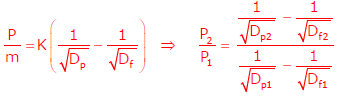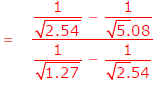P2/100 = 0.707, P2= 70.7kW

*Answer can only contain numeric values
QUESTION: 45

Calculate the heat required (in kJ, up to 1 digit after the decimal point) to raise the temperature of 1 mole of a solid material from 100°C to 1000°C. The specific heat ( CP ) of the material (in J/mol-K) is expressed as CP = 20 + 0.005T, where T is in K. Assume no phase change.______

Important : you should answer only the numeric value

Solution: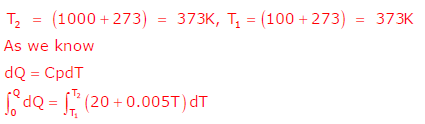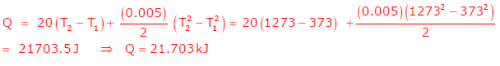QUESTION: 46

In a double pipe counter-current heat exchanger, the temperature profiles shown in the figure were observed. During operation, due to fouling inside the pipe, the heat transfer rate reduces to half of the original value. Assuming that the flow rates and the physical properties of the fluids do not change, the LMTD (in °C) in the new situation is

Solution: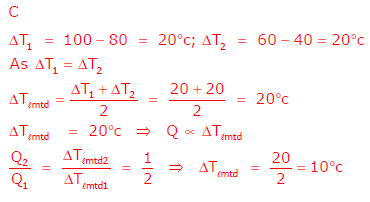*Answer can only contain numeric values
QUESTION: 47

The vapor-liquid equilibrium curve of a binary mixture A-B, may be approximated
by a linear equation over a narrow range of liquid mole fractions ( 0.2 < xA <0.3)
as follows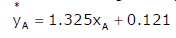Here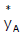is the mole of fraction of A in the vapor. 100 moles of a feed ( xA,F = 0.28) is batch distilled to a final residue ( xA,W = 0.28) . Using the Rayleigh equation, the number of moles of the residue left behind in the distillation unit, up to 2 digits after the decimal point, is __________

Important : you should answer only the numeric value

Solution: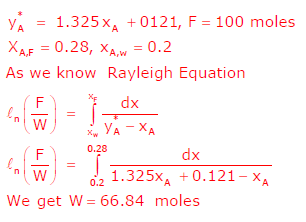QUESTION: 48

A crosscurrent cascade of N ideal stages is used to treat a feed stream of molar flow rate E. The feed stream contains a solute which is to be recovered by a pure solvent having a molar flow rate S. The solvent is divided equally between these N stages. The linear equilibrium curve relating the mole fractions x and y* of the solute in the raffinate and the extract respectively, is given by y* = m x. Assume dilute conditions. The ratio of the solute mole fraction in the original feed to that in the exit raffinate stream i.e. ( x0 / xN )is given by

Solution:

For Cross Current Cascade Equilibrium relation is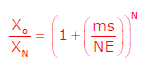*Answer can only contain numeric values
QUESTION: 49

A study was conducted in which water was pumped through cylindrical pipes made of a sparingly soluble solid. For a given pipe and certain flow conditions, the mass transfer coefficient kc has been calculated as 1 mm/s using the correlation; Sh = 0.025 Re0.6 Sc0.33 .If the velocity of the fluid and the diameter of the pipe are both doubled, what is the new value of kc in mm/s, up to 2 digits after the decimal point? ______

Important : you should answer only the numeric value

Solution: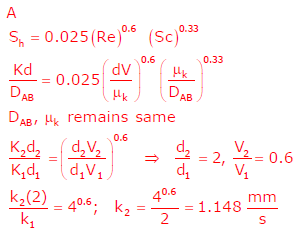*Answer can only contain numeric values
QUESTION: 50

The gas phase decomposition of azomethane to give ethane and nitrogen takes
place according to the following sequence of elementary reactions.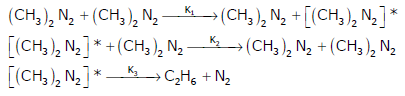Using the pseudo-steady-state-approximation for [(CH3)2N2]*, the order with
respect to azomethane in the rate expression for the formation of ethane, in the limit of high concentrations of azomethane, is ______________

Important : you should answer only the numeric value

Solution: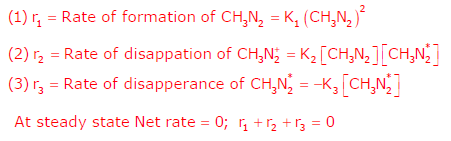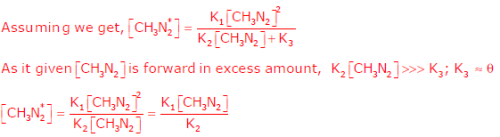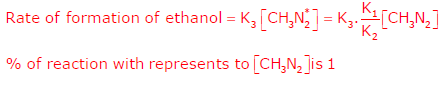*Answer can only contain numeric values
QUESTION: 51

A first order liquid phase reaction is carried out isothermally at a steady state in a CSTR and 90% conversion is attained. With the same inlet conditions and for the same overall conversion, if the CSTR is replaced by two smaller and identical isothermal CSTRs in series, the % reduction in total volume, to the nearest integer, is _____________

Important : you should answer only the numeric value

Solution: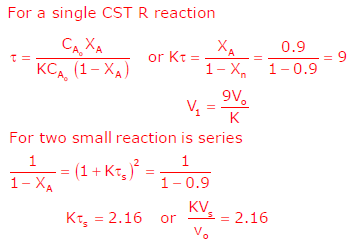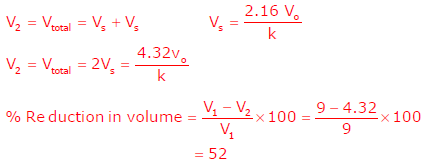QUESTION: 52

Match the reactant-product combination in Group 1 with the unit process in Group
2.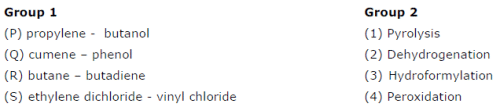Solution: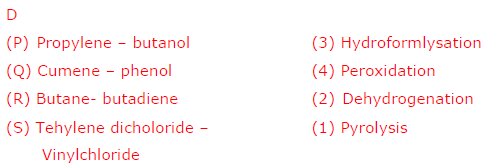QUESTION: 53

Identify which of the following statements are FALSE.
(P) Oils with an oleic radical (1 double bond) are more suitable than oils with a
linolenic radical (3 double bonds) as film forming vehicles for paints
(Q) Production of synthesis gas from coal and steam is an endothermic process
(R) Use of chlorine for bleaching of wood pulp results in the release of dioxins
(S) In the manufacture of urea from ammonia, the main intermediate product
formed is ammonium bicarbonate

Solution:

D
Ammonium carbamate is the main intermediate product during manufacturing of urea from NH3.

QUESTION: 54

A unit gain 2nd order underdamped process has a period of oscillation 1 second
and decay ratio 0.25. The transfer function of the process is

Solution: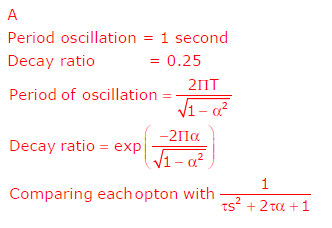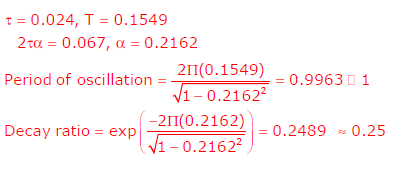*Answer can only contain numeric values
QUESTION: 55

A control valve, with a turndown ratio of 50, follows equal percentage characteristics. The flow rate of a liquid through the valve at 40% stem position is 1 m3/h. What will be the flow rate in m3/h at 50% stem position, if the pressure drop across the valve remains unchanged? (Up to 2 digits after the decimal
point.)

Important : you should answer only the numeric value

Solution: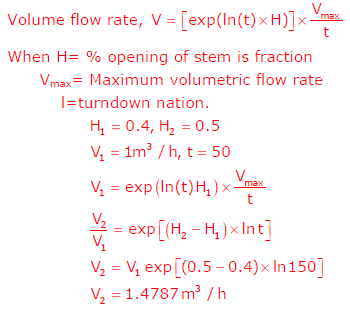*Answer can only contain numeric values
QUESTION: 56

The purchase cost of a heat exchanger of 20 m2 area was Rs. 500000 in 2006. What will be the estimated cost (in Rs. to the nearest integer) of a similar heat exchanger of 50 m2 area in the year 2013? Assume the six-tenths factor rule for scaling and the cost index for 2006 as 430.2. The projected cost index for the
year 2013 is 512.6.

Important : you should answer only the numeric value

Solution: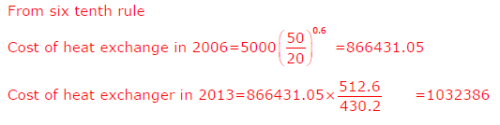*Answer can only contain numeric values
QUESTION: 57

A plant manufactures compressors at the rate of N units/day. The daily fixed charges are Rs. 20000 and the variable cost per compressor is Rs. 500 + 0.2 N1.3. The selling price per compressor is Rs. 1000. The number of compressors to be manufactured, to the nearest integer, in order to maximize the daily profit is

Important : you should answer only the numeric value

Solution:

Daily profit = selling price – cost price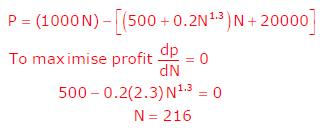*Answer can only contain numeric values
QUESTION: 58

Common Data Questions: 58 and 59

A reverse osmosis unit treats feed water (F) containing fluoride and its output consists of a permeate stream (P) and a reject stream (R). Let CF, CP, and CR denote the fluoride concentrations in the feed, permeate, and reject streams, respectively. Under steady state conditions, the volumetric flow rate of the reject is 60 % of the volumetric flow rate of the inlet stream, and CF = 2 mg/L and CP =0.1 mg/L.

Q.

The value of CR in mg/L, up to one digit after the decimal point, is

Important : you should answer only the numeric value

Solution: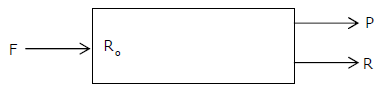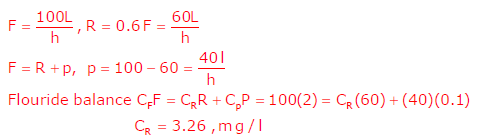*Answer can only contain numeric values
QUESTION: 59

A reverse osmosis unit treats feed water (F) containing fluoride and its output consists of a permeate stream (P) and a reject stream (R). Let CF, CP, and CR denote the fluoride concentrations in the feed, permeate, and reject streams, respectively. Under steady state conditions, the volumetric flow rate of the reject is 60 % of the volumetric flow rate of the inlet stream, and CF = 2 mg/L and CP =0.1 mg/L

Q.

A fraction f of the feed is bypassed and mixed with the permeate to obtain treated water having a fluoride concentration of 1 mg/L. Here also the flow rate of the reject stream is 60% of the flow rate entering the reverse osmosis unit (after the bypass). The value of f, up to 2 digits after the decimal point, is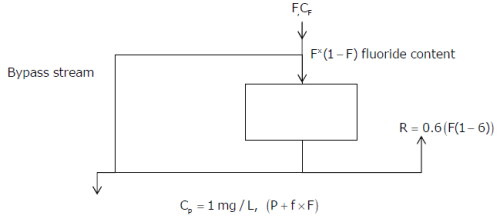Important : you should answer only the numeric value

Solution:

Mass balance at Reverse osmosis in and out
P=0.4(1-f)F
Mass balance of fluoride on bypass stream

F x  f x  2 = [ (F x f)+ P]
F x  f x  2 = [ (F x f) + 0.4 (1- f) F]
on solving, we get

2 x f =0.4(1-f) +f
F=0.4/1.4 =0.286

*Answer can only contain numeric values
QUESTION: 60

Common Data Questions: 60 and  61

Liquid reactant A decomposes as follows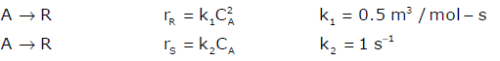An aqueous feed of composition CA0 = 30 mol/m3, CR0 = 2 mol/m3, and CS0= 1 mol/m3 enters a CSTR in which the above reactions occur. Assume isothermal and steady state conditions.

Q.

If the conversion of A is 80 %, the concentration of R in the exit stream in
mol/m3, to the nearest integer, is______

Important : you should answer only the numeric value

Solution: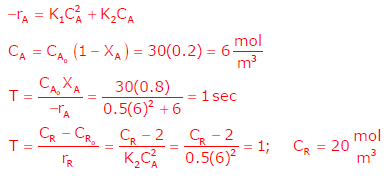*Answer can only contain numeric values
QUESTION: 61

Liquid reactant A decomposes as followsAn aqueous feed of composition CA0 = 30 mol/m3, CR0 = 2 mol/m3, and CS0= 1 mol/m3 enters a CSTR in which the above reactions occur. Assume isothermal and steady state conditions.

Q.

What is the % conversion of A, to the nearest integer, so that the concentration of S in the exit stream is 11.8 mol/m3?

Important : you should answer only the numeric value

Solution: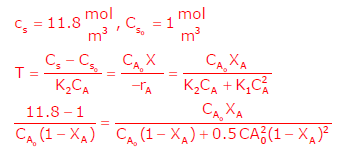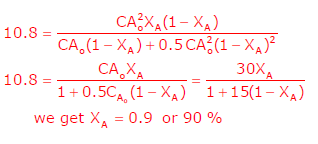QUESTION: 62

The vapor liquid equilibrium relation for an ideal binary system is given by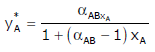Here xA and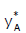are the mole fractions of species A in the liquid and vapor, respectively. The relative volatility ( αAB)  is greater than unity.

Q.

The liquid mole fraction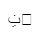at which the maximum difference between the equilibrium vapor mole fraction and liquid mole fraction occurs is

Solution: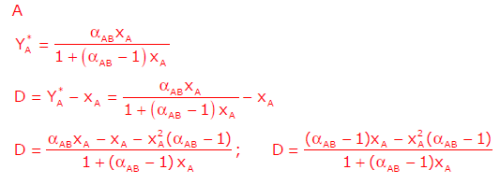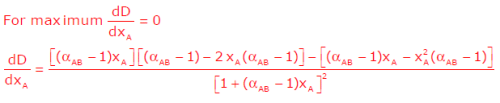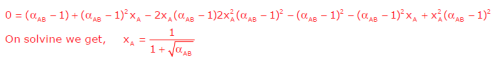QUESTION: 63

The vapor liquid equilibrium relation for an ideal binary system is given byHere xA andare the mole fractions of species A in the liquid and vapor, respectively. The relative volatility ( αAB)  is greater than unity.

Q.

A liquid having the composition found in the first part of the linked answer question, is flash distilled at a steady state to a final liquid mole fraction of 0.25. If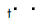is 2.5, the fraction of the feed vaporized is

Solution:
QUESTION: 64

Consider the following transfer function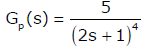(Note: The unit of the process time constant is in seconds.)

Q.

The crossover frequency (in rad/s) of the process is

Solution: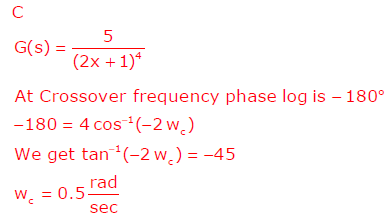QUESTION: 65

Consider the following transfer function(Note: The unit of the process time constant is in seconds.)

Q.

For the computation of Ziegler-Nichols settings, the ultimate period (in s/cycle)
and the ultimate gain are

Solution: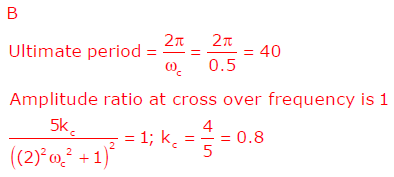Use Code STAYHOME200 and get INR 200 additional OFF Use Coupon Code